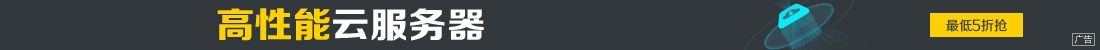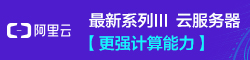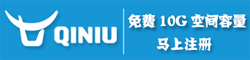# php中的三元运算符使用说明

••echo
\$a == 1 ? 'one' :
\$a == 2 ? 'two' :
\$a == 3 ? 'three' :
\$a == 4 ? 'foura' : 'other';
echo "\n";

<BR>

echo (\$a == 1 ? 'one' :
( \$a == 2 ? 'two' :
( \$a == 3 ? 'three' :
(\$a == 4 ? 'four' : 'other'))));

<!--?php
// 乍看起来下面的输出是 'true'
echo (true?'true':false?'t':'f');

// 然而，上面语句的实际输出是't'，因为三元运算符是从左往右计算的

// 下面是与上面等价的语句，但更清晰
echo ((true ? 'true' : 'false') ? 't' : 'f');

echo (
(((\$a == 1 ? 'one' :
\$a == 2) ? 'two' :
\$a == 3 )? 'three' :
\$a == 4 )? 'four' : 'other');

\$a==1=>false=>\$a==2?true=>'two'=true=>'three'=true=>'four'